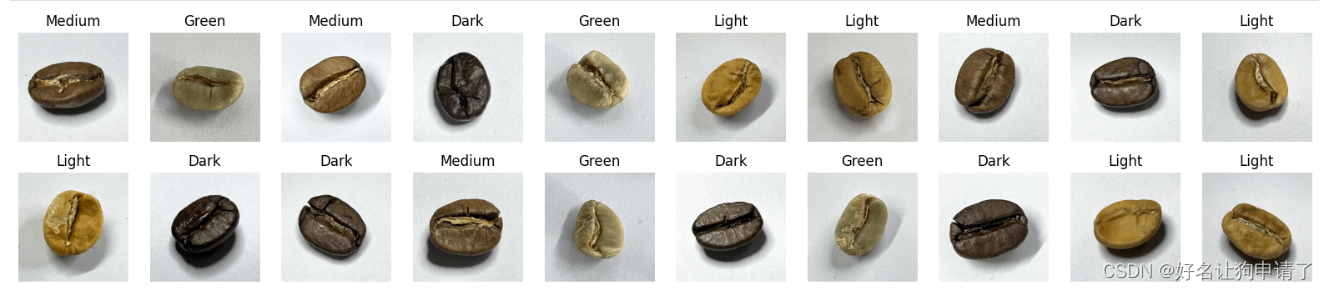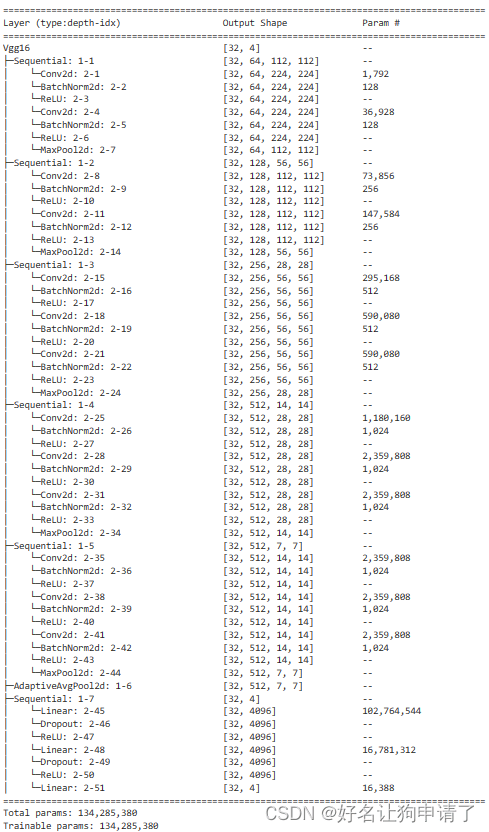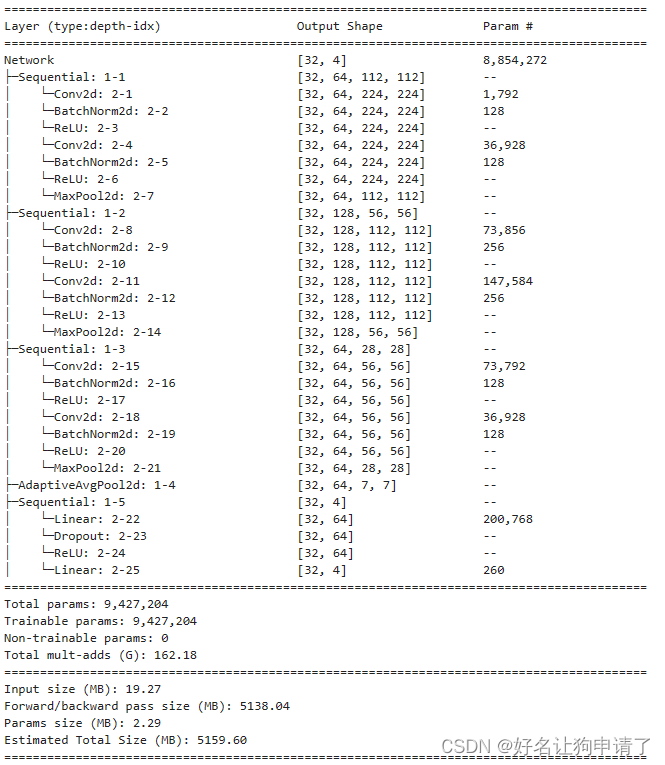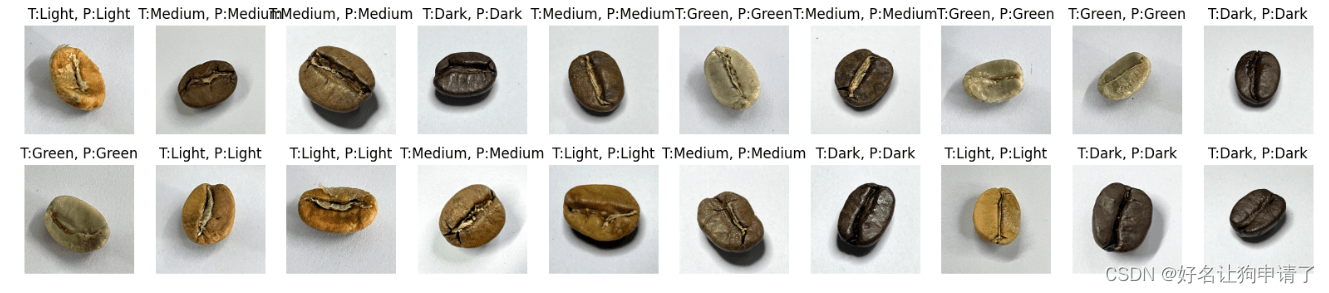# Convolutional neural network implements coffee bean classification – P7

• Original author: Classmate K | Tutoring, project customization
• Article source: Student K’s study circle

#### Directory

• environment
• step
• Environment settings
• package reference
• global device object
• data preparation
• View image information
• Make a dataset
• Model design
• Manually built vgg16 network
• Streamlined coffee bean identification network
• Model training
• Write training function
• Write test function
• Start training
• Show training process
• Model effect display
• Summary and experience

## Environment

• System: Linux
• Language: Python3.8.10
• Deep learning framework: Pytorch2.0.0 + cu118
• Graphics card: A5000 24G

## Steps

### Environment settings

#### Package reference

```import torch
import torch.nn as nn # network
import torch.optim as optim # optimizer
from torch.utils.data import DataLoader, random_split #Data set division

import pathlib, random, copy # Folder traversal to implement model deep copy
from PIL import Image # python’s own image class
import matplotlib.pyplot as plt # chart
import numpy as np
from torchinfo import summary #Print model parameters
```

#### Global device object

Conveniently copy models and data to the target device

```device = torch.device('cuda' if torch.cuda.is_available() else 'cpu')
```

### Data preparation

#### View image information

```data_path = 'coffee_data'
data_lib = pathlib.Path(data_path)
coffee_images = list(data_lib.glob('*/*'))

# Print information about 5 images
for _ in range(5):
image = random.choice(coffee_images)
print(np.array(Image.open(str(image))).shape)
```From the printed information, we can see that the size of the images are all 224×224, which is an image size often used by CV, so I will not use Resize to scale the image later.

```# Print 20 images for a rough look
plt.figure(figsize=(20, 4))
for i in range(20):
plt.subplot(2, 10, i + 1)
plt.axis('off')
image = random.choice(coffee_images) # Randomly select an image
plt.title(image.parts[-2]) # Get its folder name through the glob object, which is the category name
plt.imshow(Image.open(str(image))) # Display
```Through the display, you can have a general understanding of the images in the data set.

#### Create a data set

First write the data preprocessing process to load the images in the folder using pytorch’s API

```transform = transforms.Compose([
transforms.ToTensor(), # First convert the image into a tensor
transforms.Normalize( # Normalize the pixel values and set the data range to -1,1
mean=[0.485, 0.456, 0.406],
std=[0.229, 0.224, 0.225],
),
])
```

```dataset = datasets.ImageFolder(data_path, transform=transform)
```

Get all category names from the data

```class_names = [k for k in dataset.class_to_idx]
print(class_names)
```Divide the data set into a training set and a validation set

```train_size = int(len(dataset) * 0.8)
test_size = len(dataset) - train_size

train_dataset, test_dataset = random_split(dataset, [train_size, test_size])
```

Divide the dataset into batches to use mini-batch gradient descent

```batch_size = 32
```

### Model design

At the beginning, I created the Vgg-16 network manually and found that the model converged after a few iterations, so I started to streamline the model.

#### Manually built vgg16 network

```class Vgg16(nn.Module):
def __init__(self, num_classes):
super().__init__()
\t\t
self.block1 = nn.Sequential(
nn.BatchNorm2d(64),
nn.ReLU(),
nn.BatchNorm2d(64),
nn.ReLU(),
nn.MaxPool2d(2),
)
self.block2 = nn.Sequential(
nn.BatchNorm2d(128),
nn.ReLU(),
nn.BatchNorm2d(128),
nn.ReLU(),
nn.MaxPool2d(2),
)
self.block3 = nn.Sequential(
nn.BatchNorm2d(256),
nn.ReLU(),
nn.BatchNorm2d(256),
nn.ReLU(),
nn.BatchNorm2d(256),
nn.ReLU(),
nn.MaxPool2d(2),
)
self.block4 = nn.Sequential(
nn.BatchNorm2d(512),
nn.ReLU(),
nn.BatchNorm2d(512),
nn.ReLU(),
nn.BatchNorm2d(512),
nn.ReLU(),
nn.MaxPool2d(2),
)
self.block5 = nn.Sequential(
nn.BatchNorm2d(512),
nn.ReLU(),
nn.BatchNorm2d(512),
nn.ReLU(),
nn.BatchNorm2d(512),
nn.ReLU(),
nn.MaxPool2d(2),
)
self.classifier = nn.Sequential(
nn.Linear(7*7*512, 4096),
nn.Dropout(0.5),
nn.ReLU(),
nn.Linear(4096, 4096),
nn.Dropout(0.5),
nn.ReLU(),
nn.Linear(4096, num_classes),
)

def forward(self, x):
x = self.block1(x)
x = self.block2(x)
x = self.block3(x)
x = self.block4(x)
x = self.block5(x)
x = self.pool(x)
x = x.view(x.size(0),-1)
x = self.classifier(x)
return x
vgg = Vgg16(len(class_names)).to(device)
summary(vgg, input_size=(32, 3, 224, 224))
```By printing the model structure, we can find that the VGG-16 network has a total of 134285380 trainable parameters (I added BatchNorm, which is slightly more than the official one). The amount of parameters is very huge. For a small scene such as coffee bean identification, so Multiple trainable parameters are definitely a waste, so the original VGG-16 network structure was streamlined.

#### Simplified coffee bean identification network

```class Network(nn.Module):
def __init__(self, num_classes):
super().__init__()
\t\t
self.block1 = nn.Sequential(
nn.BatchNorm2d(64),
nn.ReLU(),
nn.BatchNorm2d(64),
nn.ReLU(),
nn.MaxPool2d(2),
)

self.block2 = nn.Sequential(
nn.BatchNorm2d(128),
nn.ReLU(),
nn.BatchNorm2d(128),
nn.ReLU(),
nn.MaxPool2d(2),
)
\t\t
self.block3 = nn.Sequential(
nn.BatchNorm2d(64),
nn.ReLU(),
nn.BatchNorm2d(64),
nn.ReLU(),
nn.MaxPool2d(2),
)

self.classifier = nn.Sequential(
nn.Linear(7*7*64, 64),
nn.Dropout(0.4),
nn.ReLU(),
nn.Linear(64, num_classes)
)
\t
def forward(self, x):
x = self.block1(x)
x = self.block2(x)
x = self.block3(x)
x = self.pool(x)
x = x.view(x.size(0), -1)
x = self.classifier(x)
return x
model = Network(len(class_names)).to(device)
summary(model, input_size=(32, 3, 224, 224))
```It can be seen that the number of parameters of the streamlined network model is less than 1/10 of the original, but its accuracy on the test set can still reach 100%!

### Model training

#### Write training function

```def train(train_loader, model, loss_fn, optimizer):

train_loss, train_acc = 0, 0
x, y = x.to(device), y.to(device)
\t
pred = model(x)
loss = loss_fn(pred, y)

loss.backward()
optimizer.step()

train_loss + = loss.item()
train_acc + = (pred.argmax(1) == y).type(torch.float).sum().item()

train_loss /= num_batches
train_acc /= size

return train_loss, train_acc
```

#### Write test functions

```def test(test_loader, model, loss_fn):
\t
test_loss, test_acc = 0, 0
x, y = x.to(device), y.to(device)

pred = model(x)
loss = loss_fn(pred, y)

test_loss + = loss.item()
test_acc + = (pred.argmax(1) == y).type(torch.float).sum().item()

test_loss /= num_batches
test_acc /= size

return test_loss, test_acc
```

#### Start training

First define the loss function, and the optimizer sets the learning rate. Here we also get the attenuation of the learning rate, plus the total number of iterations, and the storage location of the best model.

```epochs = 30
loss_fn = nn.CrossEntropyLoss()
scheduler = optim.lr_scheduler.LambdaLR(optimizer=optimizer, lr_lambda=lambda epoch: 0.92**(epoch//2))
best_model_path = 'best_coffee_model.pth'
```

Then write a training + test loop and record the data during the training process

```best_acc = 0
train_loss, train_acc = [], []
test_loss, test_acc = [], []
for epoch in epochs:
model.train()
epoch_train_loss, epoch_train_acc = train(train_loader, model, loss_fn, optimizer)
scheduler.step()

model.eval()
epoch_test_loss, epoch_test_acc = test(test_loader, model, loss_fn)
\t
train_loss.append(epoch_train_loss)
train_acc.append(epoch_train_acc)
test_loss.append(epoch_test_loss)
test_acc.append(epoch_test_acc)

lr = optimizer.state_dict()['param_groups']['lr']

if best_acc < epoch_test_acc:
best_acc = epoch_test_acc
best_model = copy.deepcopy(model)

print(f"Epoch: {<!-- -->epoch + 1}, Lr:{<!-- -->lr}, TrainAcc: {<!-- -->epoch_train_acc*100:.1f} , TrainLoss: {<!-- -->epoch_train_loss:.3f}, TestAcc: {<!-- -->epoch_test_acc*100:.1f}, TestLoss: {<!-- -->epoch_test_loss:.3f} ")

print(f"Saving Best Model with Accuracy: {<!-- -->best_acc*100:.1f} to {<!-- -->best_model_path}")
torch.save(best_model.state_dict(), best_model_path)
print('Done')
```It can be seen that the accuracy of the model on the test set reaches up to 100%.

#### Show the training process

```epoch_ranges = range(epochs)
plt.figure(figsize=(20,6))
plt.subplot(121)
plt.plot(epoch_ranges, train_loss, label='train loss')
plt.plot(epoch_ranges, test_loss, label='validation loss')
plt.legend(loc='upper right')
plt.title('Loss')

plt.figure(figsize=(20,6))
plt.subplot(122)
plt.plot(epoch_ranges, train_acc, label='train accuracy')
plt.plot(epoch_ranges, test_acc, label='validation accuracy')
plt.legend(loc='lower right')
plt.title('Accuracy')
```### Model effect display

```model.load_state_dict(torch.load(best_model_path))
model.to(device)
model.eval()

plt.figure(figsize=(20,4))
for i in range(20):
plt.subplot(2, 10, i + 1)
plt.axis('off')
image = random.choice(coffee_images)
input = transform(Image.open(str(image))).to(device).unsqueeze(0)
pred = model(input)
plt.title(f'T:{<!-- -->image.parts[-2]}, P:{<!-- -->class_names[pred.argmax()]}')
plt.imshow(Image.open(str(image)))
```It can be seen from the results that indeed all coffee beans have been correctly identified.

## Summary and experience

• Because the current network is still quickly converging to a very high level, there should be a lot of room for simplification, but some accuracy may be slightly sacrificed.
• The selection of the model should be determined based on the actual task. For tasks such as coffee bean type identification, using VGG-16 is too wasteful.
• During the streamlining process, no obvious change in training speed was felt, indicating that there is no direct correlation between parameter quantity and training speed.
• Consecutive multi-layer convolution operations with the same parameters seem to have better results than using only one layer.